Contents

Introduction
The NEFCON Model - Introduction

Introduction

This document is an Online User's Guide to the neuro-fuzzy model NEFCON implementation under MATLAB/SIMULINK. It can be used as an introduction to system creation and as a reference guide during system modification.

The first section describes how to build up easily a learning environment for a dynamic system. The following sections describe all parts of the application in detail. Besides hints for optimal system usage are given.

If you use the MATLAB HTML-Browser HTHELP you will be able to start the GUI-Tools directly out of this documentation. Moreover, if you are using the GUI-Tools, you will be able to 'jump' into any part of this documentation by pressing the '?' or 'HELP' button. If you are using a standard HTML-Browser, screenshots of the GUI-Tools will be embedded into this document.

The NEFCON-Model was originally developed by the research group Fuzzy Systems at the Technical University of Braunschweig, Department of Computer Science, Germany.

It is a neuro-fuzzy controller based on a generic fuzzy-perceptron. It is able to learn fuzzy sets and rules by a reinforcement learning algorithm.

Contents Index

NEFCON System creation

Creation of a New Learning Environment

To create a new learning environment for a new system that should be controlled follow these steps:
1. Copy the SIMULINK-block 'NEFCON System' (see model nb.m or any sample in directory samples) into your SIMULINK Model.
2. Double click on the block to start the 'NEFCON Control' GUI-Tool.
3. Press the Create button (the GUI-Tool 'Create Settings' come up).
4. Select the Signal-Generator you want to use and select NEFCON Learning System.
5. Set the correct number of inputs. (See 'Learning Algorithm' and 'Error Definition' if you are unsure.)
6. Press the Create button.
7. Connect the SIMULINK-Blocks to your system.
8. If you want to use automatic saving of simulation results, connect SIMULINK To Workspace blocks to your system and fill vector nef_u and nef_y (see sample below).
9. Check gains and intervals in 'Input Preprocessing'.
10. In 'NEFCON Control' enter simulation time and step size.
11. Press the Start button.
The NEFCON-Model now learns to control your system.

A detailed description of the learning process and parameter modification is described in Section 'Learning Algorithm'. Hints for manual definition of the rulebase are described in 'Setting up a Rulebase'.

A sample of a created learning environment is shown below.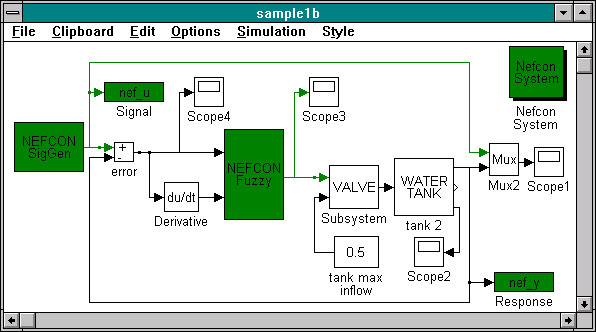Start SIMULINK sample: sample1b.m.

Contents Index

NEFCON \ NEFCON Control

NEFCON Control

The NEFCON Control is the main window to control the settings and execution of the NEFCON learning algorithm.

You can change to basic parts of the NEFCON-System by pressing one of the four big buttons:

Besides, the actual simulation status of the system is shown and can be controlled. To control execution use the Simulate/Continue and Stop buttons. You can also skip or repeat learning steps using the buttons '<<' and '>>' (use careful). In particular you can speed up optimizing by switching between control and optimize in ‘good’ and ‘bad’ control periods. Learning steps can be disabled with the checkboxes in the bottom row.

To create a new learning environment press the Create button. See 'Creation of a new Learning Environment' for details.

The actual settings are automatically loaded, and saved in a file named name.NEF, where name is the name of your current SIMULINK system file. If you do not want to save the current settings double click on the Window GUI-Close, do not use the Close button or the menu Close item. (Due to a system bug its not possible to start a dialog box after pressing a button.)

The entries in the File menu can be used to load/save a fismatrix or the complete NEFCON settings (including fismatrix). Also, you can have a look at old simulation results using Show Loops.

Contents Index

NEFCON \ learning algorithm

Learning Algorithm

The parameters of the learning algorithms can be modified using this GUI-Tool.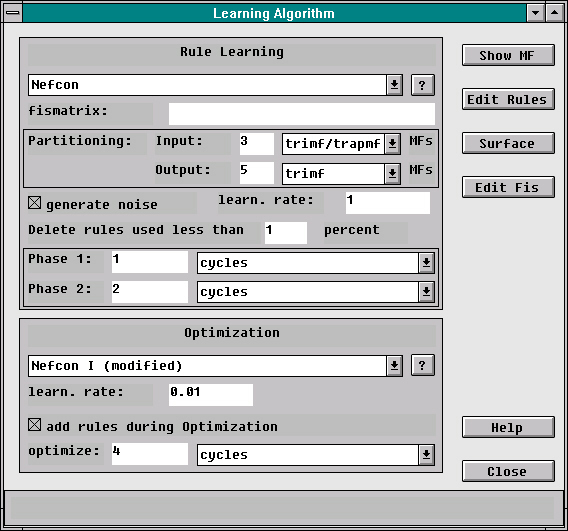Start GUI-Tool:
nefgui_o.m.

The learning algorithm can be separated into the two main parts rule learning and optimization. The options for this parts are described in the Sections 'Rule Learning Options' and 'Optimization Options'.

By pressing any of the four buttons in the upper right corner you can view or edit the current fismatrix. This can be done even during simulation.

Contents Index

Rule Learning Options

The Learning Algorithm

1. Bottom-Up Learning
The fuzzy sets for input and output will be initialized with equally distributed membership functions. The type and number of initial membership functions can be selected in the box below. The rulebase is empty. If a
fismatrix is defined in the field below, the membership functions and rules will be used as initial data. (See 'Setting up a Rulebase' for details.) Rules will be added during the learning process.
2. NEFCON
The fuzzy sets for input and output will be initialized with equally distributed membership functions. The type and number of initial membership functions can be selected in the box below. A full set of rules will be set up before learning. If a fismatrix is defined in the field below the membership functions and rules will be used as initial data. (See 'Setting up a Rulebase' for details.) If the rulebase is empty a full set of rules will be added. During the learning process, 'bad' rules will be deleted.
3. Disabled - Initial Fismatrix
Rule learning is disabled. A valid initial fismatrix filename must be entered in the box below.
4. Disabled - Current Fismatrix
Rule learning is disabled. The current fismatrix will be used as initial data.

Generate Noise during Rule Learning
If NEFCON-Signal-Generators are used this option enables 40% noise during rule learning. This will enforce optimal rule setting (neural connections generation) and coverage of system states. The global boolean variable NEFCON_NOISE will be used to turn noise generation on and off. So you will be able to use the variable in your own noise generation tools.

Learning Rate
If you are using the bottom-up learning algorithm, you can enter the learning rate in this field. Normally you should use a rate between 0.5 and 2.
If you use the NEFCON learning algorithm, the rate define the relative size of an interval [-d,d] for the error signal. Rules causing an error in this range are no deleted during phase 1. Normally you should use a rate between 0.05 and 0.3.

Delete rules used less than ... percent
Rules, which will be used less than X percent, will be removed after rule learning phase 2.

In the box below you can enter the duration of rule learning phase 1 and 2.

Contents Index

Optimization Options

The Optimization Method

1. NEFCON I
The modified original NEFCON learning algorithm will be used for optimizing fuzzy sets. (mamdani controller; shifting of fuzzy sets in conclusion, modifying size of base of antecedents)
2. NEFCON II
A new NEFCON learning algorithm will be used. (mamdani controller; shifting of all fuzzy sets)
3. disabled
Optimizing is disabled. This learning step will be skipped.

Add Rules during Optimization
This option must be used if you use the bottom-up rule learning algorithm and the system space is not covered during rule learning. This is critical for systems which should be controlled by a PID-controller (integral part), because zero output will be forced for undefined conditions.

In the box below you can enter the duration of optimizing.

Contents Index

NEFCON \ Error Definition

Error Definition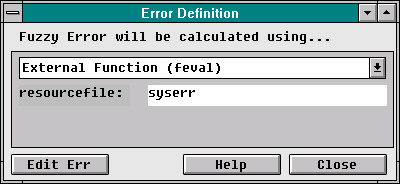Start GUI-Tool:
nefgui_e.m.

The fuzzy error can be defined by using a fismatrix, input1 or a MATLAB function. The name of the external resource can be typed below. The resource can be edited by pressing the Edit Err button.

Using a FISMATRIX
You use a fismatrix, if you want to define the error by a fuzzy inference system.

Using Input1 as Error
You can use a input1 for error definition. Usually input1 is the difference between value and current response.

Bounded error calculation
You use the bounds of the NEFCON bounded signal generator to calculate the error. Input1 must be the difference between value and current response.

Using a MATLAB Function
You can use a m-function with the following parameters for error definition:
function ferr = FNAME(input, bounds)
Input will be the vector of input signals. If you use the bounded signal generator, bounds will be a vector containing the bounds and the original signal. The function must return the error signal in [-1,1]. Sample function included: syserr.m.

Contents Index

NEFCON \ Input Preprocessing

Input Preprocessing

The parameters of input preprocessing, and to reduce the number of dialogs for system creation, the parameters of output postprocessing, can be modified using this GUI-Tool.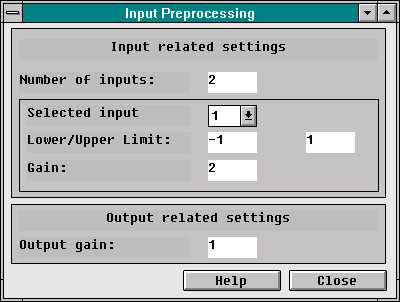Start GUI-Tool:
nefgui_i.m.

The following settings can be changed in this dialog:

Number of inputs:
The number of input signals to the NEFCON-System.

For every input you can change the following settings by selecting the number of input:

Upper/lower limit: The interval to be used for controlling.

Gain: The gain to be used for controlling.

For output only the gain can be modified.

Hints for Selecting Input Signals

The difference between optimal value and current response of the system always must be used as an input signal. In most cases you also have to use the derivative (also for error calculation), because fuzzy controllers only use static information. If the system has to get different control signals for the same difference signal (e.g. difference is zero but the system needs a bias value to stay at 1 and no bias to stay at zero) then you must use the current value, response or an integral part as input too.

Contents Index

NEFCON \ Create Settings

Create Settings

The settings used for system creation can be changed in this dialog box.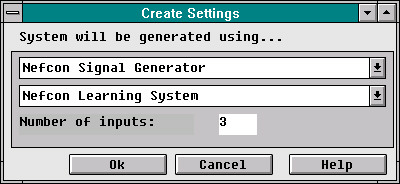Start GUI-Tool:
nefgui_c.m.

Using this GUI-Tool you can select the signal generator. See NEFCON Signal Generators for details. Also, you can select to create an environment using the NEFCON learning algorithm or a standard fuzzy controller. Besides, you can change the number of inputs of the selected system.

Contents Index

NEFCON \ Fismatrix

Fismatrix

The fismatrix describes a complete Fuzzy Inference System (membership functions and rulebase). It is defined in the MATLAB Fuzzy Logic Toolbox and can be modified using the FIS Editor fuzzy.

See 'Fuzzy Controller' for details.

Contents Index

NEFCON \ Fuzzy Controller

Fuzzy Controller

The Fuzzy Controller is part of the MATLAB Fuzzy Logic Toolbox. To modify the current
fismatrix (membership functions, rulebase etc.) the following GUI-Tool will be used: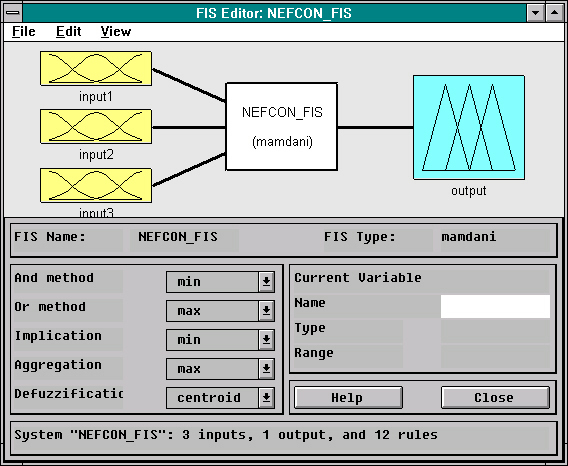Start GUI-Tool: fuzzy.m.

See printed documentation for details or use the fuzzy.m online documentation.

Hint: The methods for and, or, implication, aggregation and defuzzification shouldn't be modified. Different methods can be used, but you should be familiar with the theory of the NEFCON-model, if you change the default settings.

Contents Index

NEFCON \ Signal Generators

NEFCON Signal Generators

NEFCON bounded signal generator You can select the NEFCON bounded signal generator if you want to set up the signal manually using the mouse. Also, you can describe the error for the learning algorithm by 'fuzzy-bounds'.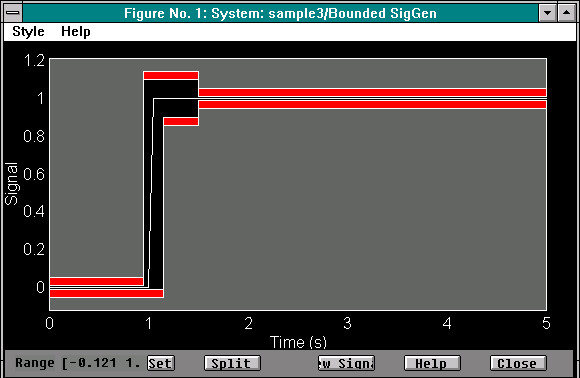This tool is the modified version of the error definition tool used in the MATLAB NCD Tool-box. It was originally written by A. Potwin, ‘The Mathworks’.
You can move the bounds and the signal by dragging it with your mouse. The signal range can be changed by entering the new interval and pressing the set button.
You can split the bars for error definition by selecting them and pressing the split button.
By pressing the New Signal button the old signal will be erased with the default signal. Besides, the time range will be changed to your actual SIMULINK settings.
The signal generator support noise generation.

NEFCON signal generator If you use the NEFCON signal generator, a block containing the SIMULINK SigGen will be used.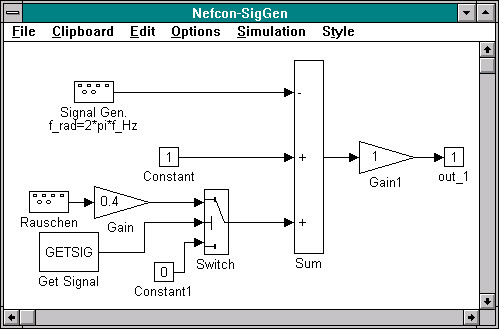The signal generator support noise generation.

Contents Index

NEFCON \ Setting up a Rulebase

Setting up a Rulebase

If you want to setup a rulebase manually to introduce previous knowledge to the system think of the following hints.

1. Define input signals.
Select the input signals of the learning system careful. See
'Hints for Selecting Input Signals'.
2. Define the input and output intervals.
The interval describing the difference between optimal value and current response of the system should always cover all states, just as the interval describing the integral part. The input interval for the derivative should be [-1,1] in most cases.
The output interval must overlap the values required for system control.
3. Partition the intervals.
You can always use triangular membership functions. Critical sections should be partitioned with smaller membership functions. At least three membership functions should be used.
4. Set up the rulebase.
The rulebase should overlap all system states.

To conveniently enter the rules and fuzzy sets use the fuzzy.m GUI-Tool of the Fuzzy Logic Toolbox.

Contents Index

NEFCON learning algorithm

Contents